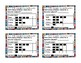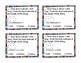# 2nd Grade Differentiated Exit Tickets Aligned to GoMath Bundle Chapters 7 - 11Subject
Resource Type
File Type
Zip (45 MB|181 pages)
\$12.25
Bundle
List Price:
\$15.75
You Save:
\$3.50
\$12.25
Bundle
List Price:
\$15.75
You Save:
\$3.50
Products in this Bundle (5)
1. If you use GoMath, this will save you a lot of time and energy. This product includes exit tickets that are aligned to each lesson in GoMath Chapter 10. There are 4 differentiated exit slips for each lesson (5 exit slips for lessons 10.4 and 10.5) Also included is a checklist with the lessons and
2. If you use GoMath, this will save you a lot of time and energy. This product includes exit tickets that are aligned to each of the 11 lessons in GoMath Chapter 11. There are 4 differentiated exit slips for each lesson. That's 44 exit tickets! Also included is a checklist with the lessons and the
3. If you use GoMath, this will save you a lot of time and energy. This product includes exit tickets that are aligned to each of the 11 lessons in GoMath Chapter 7. There are 4 differentiated exit slips for each lesson. That's 44 exit tickets! Also included is a checklist with the lessons and the
4. If you use GoMath, this will save you a lot of time and energy. This product includes exit tickets that are aligned to each lesson in GoMath Chapter 8. There are 4 differentiated exit slips for each lesson. Also included is a checklist with the lessons and the Common Core Standards. * Measure wit
5. If you use GoMath, this will save you a lot of time and energy. This product includes exit tickets that are aligned to each lesson in GoMath Chapter 9. There are 4 differentiated exit slips for each lesson (7 exit slips for lesson 9.3.) Also included is a checklist with the lessons and the Common
Bundle Description

If you use GoMath, this will save you a lot of time and energy.

This product includes exit tickets that are aligned to each of the lessons in GoMath Chapters 7 - 11.

There are 4 differentiated exit slips for each lesson. That's 181 exit tickets!

Also included is a checklist with the lessons and the Common Core Standards for each chapter.

Chapter 7: There are 4 differentiated exit slips for each lesson. That's 44 exit tickets!

* Dimes, nickels, and pennies

* Quarters, dimes, nickels, and pennies

* Count collection

* Show amounts in two ways

* One dollar

* Amounts greater than \$1

* Problem solving: money

* Time to the hour and half hour

* Time to 5 minutes

* Practice telling time

* A.M. and P.M.

Chapter 8: There are 4 differentiated exit slips for each lesson. That’s 36 exit tickets!

* Measure with inch models

* Use a ruler

* Estimate lengths in inches

* Measure with an inch ruler

* Add and subtract in inches

* Measure in inches and feet

* Estimate lengths in feet

* Choose a tool to measure

* Display measurement data in line plots

Chapter 9: There are 4 differentiated exit slips for each lesson (7 exit slips for lesson 9.3.) That’s 31 exit tickets!

* Measure with a centimeter model

* Estimate lengths in centimeters

* Measure with a centimeter ruler

* Add and Subtract lengths in centimeters (problem solving)

* Measure in centimeters and meters

* Estimate lengths in meters

* Measure and compare lengths in centimeters

Chapter 10: There are 4 differentiated exit tickets or slips for each lesson (5 exit slips for lessons 10.4 and 10.5.) That’s 26 exit tickets!

* Collect data using a tally chart

* Make picture graphs

* Make bar graphs

* Problem solving: Display data

Chapter 11: There are 4 differentiated exit tickets or slips for each lesson. That’s 44 exit tickets!

* Identify 3-dimensional shapes

* Attributes of 3-dimensional shapes

*Build a 3-dimensional shape

* Name 2-D shapes according to number of sides and vertices

* Angles in 2-D shapes

* Sort 2-D shapes according to their attributes

* Partition rectangles into equal parts

* Equal parts: halves, thirds, and fourths

* Show equal parts of a whole

* Describe equal parts of a whole

* Problem solving: Equal shares

Check out the bundle of Chapters 1 - 6 exit tickets. It's over 20% off!

GoMath:Chapters 1 - 6 Bundle Exit Tickets

Individual 2nd Grade Exit Tickets Aligned to GoMath

Total Pages
181 pages